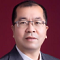As the leader in analytics, SAS provides a very rich set of SAS functions and CALL routines for programmers. There is a total of 693 uniquely named SAS functions besides the hundreds of SAS PROC steps. These SAS functions not only cover general functions such as character/string processing, mathematical, permutation & combination, probability, search and sorting, date and time, etc., but also includes a powerful domain-specific function set involving variable information and control, external files and system extensions, web services and tools, descriptive statistics, financial calculations, geodetic distance & ZIP code, Git and other fields. This article reviews the financial functions provided by the SAS system and demonstrates how to use them to do financial analysis and simplify your decisions. Functions with the same name can be invoked easily during programming with SAS DATA step, DS2, FedSQL and CAS environment, which will improve your programs' portability.

## Overview

SAS provides 94 financial functions in total, which are mainly used to calculate financial values, such as interest, periodic payments, depreciation, and prices for European options on stocks and futures. The 53 financial functions conforming to industry standards are packaged in 1 general function called FINANCE. Each finance function has a unique string identifier for the first parameter of the FINANCE function. These functions have to be invoked via the FINANCE function. For example, we can use irr=finance("IRR", -400,100,200,300); to compute the internal rate of return (IRR) for a series of cash flows.

The 53 FINANCE functions are compatible with the 53 financial functions provided by Microsoft Excel for Office 365. In fact, the list of financial functions available in the latest Excel for Office 365 software contains 56 functions. Among the 3 extra functions, PDURATION and RRI are specific functions for Excel 2013, and STOCKHISTORY is a specific function for Office 365. These 53 financial functions are designed for financial calculation such as depreciation, maturation, accrued interest, net present value, periodic savings, internal rates of return, etc. They can be categorized as investment, depreciation, Internal rate of return, financial value, and yield calculations. The complete list of SAS FINANCE functions is shown in Table 1.

 Category Subcategory Function Investment Calculation  Future Value  FV, FVSCHEDULE Payment  PMT, IPMT, PPMT, ISPMT Present Value  PV, NPV, XNPV Annual interest rate  EFFECT, NOMINAL Number of periods  NPER Depreciation  AMORDEGRC, AMORLINC, DB, DDB, SLN, SYD, VDB Internal rate of return  IRR, RATE, MIRR, XIRR Financial value and yield  Accrued/Cumulative Interest & Principal  ACCRINT, ACCRINTM, CUMIPMT, CUMPRINC Coupon days/date and Number  COUPDAYBS, COUPDAYS, COUPDAYSNC, COUPNCD, COUPNUM, COUPPCD Yield  INTRATE, ODDFYIELD, ODDLYIELD, YIELD, YIELDDISC, YIELDMAT Treasury bill  TBILLEQ, TBILLPRICE, TBILLYIELD Duration  DURATION, MDURATION Security  DISC, ODDFPRICE, ODDLPRICE, PRICE, PRICEDISC, PRICEMAT, RECEIVED Dollar Price  DOLLARDE, DOLLARFR

Table 1: 53 FINANCE XXX functions in one FINANCE function

Another 40 independently named SAS financial functions are used for investment or loan calculation, cash flow, depreciation, and pricing models. For example, we can use irr =irr(1,-400,100,200,300); to compute the internal rate of return (IRR) as a percentage for a series of cash flows.

There are 7 functions (IRR, NPV, PMT, PPMT, IPMT, CUMIPMT, CUMPRINC) whose names are the same as the industry standard FINANCE functions, but they have different sign-in results or even different calculation logic. For example, FINANCE PMT is used to calculate the periodic payment of an annuity, but the PMT function is used to calculate the periodic payment for a constant payment loan or the periodic savings for a future balance. Annuity and periodic savings are different in anchoring market interest rates, interest calculation, liquidity, and binding, so the same arguments return different results for them. The list is shown in Table 2.

 Category Function Investment/Loan  IRR/INTRR, NPV/NETPV, SAVING, SAVINGS, NOMRATE, EFFRATE, COMPOUND, PMT, PPMT, IPMT, CUMIPMT, CUMPRINC, MORT, TIMEVALUE Depreciation  DACCDB, DACCDBSL, DACCSL, DACCSYD, DACCTAB DEPDB, DEPDBSL, DEPSL, DEPSYD, DEPTAB Cash flow  CONVX, CONVXP, DUR, DURP, PVP, YIELDP Pricing Models  Stocks: BLKSHCLPRC/BLKSHPTPRC, GARKHCLPRC/GARKHPTPRC, MARGRCLPRC/MARGRPTPRC Futures: BLACKCLPRC/BLACKPTPRC

Table 2: 40 uniquely named financial functions

To keep semantic consistency, these industry-standard financial functions usually have the same parameter names, and some of the most important parameters are:

• Interest rate (rate): The interest rate or discount rate of an investment or loan.
• Number of periods (nper): The total number of payment periods for investment or loan.
• Payment (pmt): The regular payment that is made each period for investment or loan, the value cannot change over the life of the annuity. Typically, it contains principal and interest, but not other fees and taxes.
• Present value (pv): The current value of an investment or loan. The present value of an investment is the value at the beginning of the investment period, and the present value of the loan is the value of the principal borrowed.
• Future value (fv): The value of an investment or loan after all payments have occurred, or a cash balance that you want to attain after the last payment is made.
• Payments due Type (type): A flag indicates if payments are due at the beginning of the period. The omitted type or 0 means payments are due at the end of the period, 1 indicates payments are due at the beginning of the period.
• Period (period): Specify the period for interest rate or depreciation calculation. The period must be between 1 and the number of periods (nper).
• Day count basis (basis): Optional, specify the type of day count basis to use. 0-represents US (NASD) 30/360, default; 1-represents Actual/actual; 2-represents Actual /360; 3-represents Actual /365; 4-represents European 30/360.
• Frequency (frequency): Specify the number of coupon payments per year, 1-annual payment; 2-semiannual payment; 4-quarterly payment.

Below, we use some simple examples to show how to use these SAS financial functions to calculate and analyze mortgages.

## Mortgage calculation

The average interest rate of first home mortgage in China is 5.46% since September 2021, and the average interest rate of second home mortgages is 5.83%, up 23 and 29 basis points respectively from 2020. Assuming that the first housing loan amount is 2 million yuan (CNY), the loan periods is 25 years. If the mortgage type is Constant Payment Mortgage (CPM), how much will the monthly payment and total payment be?

The FINANCE PMT function can be used to calculate the periodic payment of an annuity, it can be used to calculate the constant payment for Constant Payment Mortgage by default. That is, the payment is a constant for all periods, the same amount of the loan, including principal and interest, is repaid every month. The required parameters of the FINANCE PMT function are the interest rate (rate), the number of periods (nper), and the loan amount as the present value (pv). The optional parameters are the future value (fv) and the payment due type (type).

The FINANCE PPMT function can be used to calculate the payment on the principal during a given period for an investment or a loan based on a constant periodic payment and a constant interest rate. The required parameters are interest rate (rate), the payment period (period), number of periods (nper), and the loan amount as the present value (pv), optional parameters are future value (fv) and payment due type (type). Since FINANCE PPMT returns the monthly principal, we can calculate the monthly interest and the principal balance (pb) that need to be repaid. The complete SAS program is as follows:

``` /*Mortgage: Constant Payment Mortgage*/ data Mortgage1; rate=0.0546/12; /*Interest rate: convert yearly 5.46% to monthly*/ nper=25*12; /*Number of periods: Term in month, convert 25 years to 300 months*/ pv=2000000; /*Present value: Loan amount - the value of the principal borrowed 2M*/   pmt = FINANCE('pmt', rate, nper, pv); /*Monthly payment*/ pb=pv; /*Principal Balance*/ do period = 1 to nper; ppmt=FINANCE('ppmt', rate, period, nper, pv); /*Payment on the principal*/ mi=pmt- ppmt; /*Payment on the interest*/ pb=pb+ppmt; output; end;   label rate="Interest Rate (monthly)" nper="Number of periods (month)" pv="Loan amount" period="Period" pmt = "Monthly payment" ppmt="Payment on principal" mi="Payment on interest" pb="Principal balance"; run;   title 'Mortgage: Constant Payment Mortgage'; proc print label; run;```

The results are as follows (Figure 1):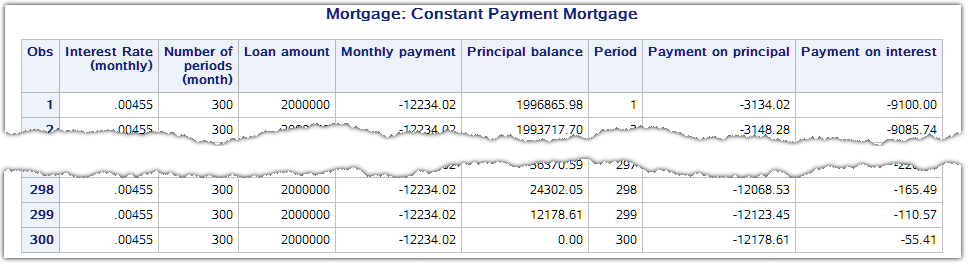Figure 1: Details of Constant Payment Mortgage payment

To show the total payment and the total interest, we use PROC REPORT to generate a summary table as Figure 2. The total payment for the Constant Payment Mortgage is 3,670,206.07, the total interest is 1,670,206.07, and the ratio of interest to principal is 83.51%.

```proc report; column period periodid pmt ppmt mi pb; format period 3.0 pmt ppmt mi pb comma15.2; define period / order noprint; define pb / order; rbreak after / summarize style=[font_weight=bold];   define periodid / computed "Period" center; compute periodid / character length=20; periodid=period ; endcomp; compute after; if _break_ in ('_RBREAK_') then periodid='Total'; endcomp; run;```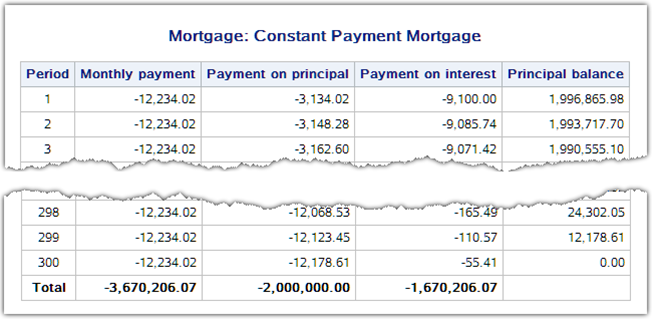Figure 2: Constant Payment Mortgage payment schedule

If the mortgage type is Constant Amortization Mortgage (CAM), what will happen to its monthly payment and interest expenses? A Constant Amortization Mortgage divides the total amount of the loan into equal parts during the payment periods and the lender repays the same amount of principal every month and the interest incurred on the remaining loan of that month. As a result, the monthly payment amount is not constant, the initial payment is high, but the interest component of the payments declines rapidly over time. For this type of mortgage, the monthly payment on the principal (ppmt) is constant; we only need to calculate the current payment on the interest (mi) generated by the principal balance (pb) to get the current total payment (pmt) for the given period. The calculation can be accomplished without calling SAS FINANCE functions. The program is as follows:

```/*Mortgage: Constant Amortization Mortgage*/ data Mortgage2; rate=0.0546/12; /*Interest rate: convert yearly 5.46% to monthly*/ nper=25*12; /*Number of periods: Term in month, convert 25 years to 300 months*/ pv=2000000; /*Present value: Loan amount - the value of the principal borrowed 2M*/   ppmt=-pv/nper; /*Monthly Payment on the principal*/ pb=pv; /*Principal Balance*/ do period=1 to nper; mi=-pb*rate; /*Payment on the interest*/ pmt=ppmt+ mi; pb=pb+ppmt; output; end;   label rate="Interest Rate (monthly)" nper="Number of periods (month)" pv="Loan amount" period="Period" pmt = "Monthly payment" ppmt="Payment on principal" mi="Payment on interest" pb="Principal balance"; RUN;   title 'Mortgage: Constant Amortization Mortgage'; PROC PRINT; RUN;```

We use the same PROC REPORT program above to generate the summary table as Figure 3. In the table, the total payment for the Constant Amortization Mortgage is 3,369,550.00, the total interest is 1,369,550.00, and the ratio of interest to principal is 68.48%. This indicates that using this type of mortgage can save 300,656.07 in interest expenses.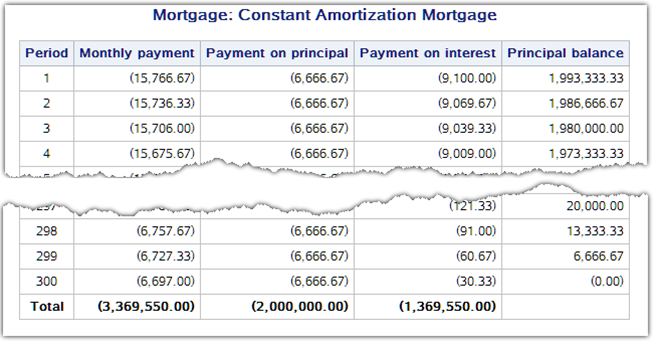Figure 3: Constant Amortization Mortgage payment schedule

To compare the monthly payment, monthly interest, and their cumulative value changes with the repayment periods for two mortgage types, we use PROC SGPANEL to draw the following figures. The code is as follows:

```/*Merge two mortgage data with type=1/2 in long format*/ data Mortgage; set Mortgage1 Mortgage2; retain type 1; if period=1 and _N_^=1 then type=type+1; pmt=abs(pmt); mi=abs(mi); ppmt=abs(ppmt); run; /*Create format for mortgage type*/ proc format; value typefmt 1="Constant Payment Mortgage" 2="Constant Amortization Mortgage"; run; data Mortgage; set Mortgage; accuppmt+ppmt; accupmt+pmt; if first.type then do; accuppmt=ppmt; accupmt=pmt; end; by type; label accuppmt="Cumulative monthly payment on principal" accupmt="Cumulative monthly payment"; format type typefmt.; run;   ods graphics / width=800px height=300px; title "Mortgage: CPM vs CAM"; proc sgpanel data=Mortgage; panelby type / novarname rows=2;   vline period / response=pmt lineattrs=(color=Cxa0a0a0); vbar period / response=pmt nooutline fillattrs=(color=cxE0E0E0) FILLTYPE=SOLID transparency= 0.5; vbar period / response=ppmt nooutline fillattrs=(color=DarkOrange) FILLTYPE=SOLID transparency= 0.5; colaxis label="Period" type=linear values=(1 to 300 by 12 ) valueattrs=(color=gray size=12pt) grid; run;   ods graphics / width=800px height=500px; title "Mortgage: CPM vs CAM"; proc sgpanel data=Mortgage; panelby type / novarname rows=2 ;   vline period / response=accupmt lineattrs=(color=Cxa0a0a0); vbar period / response=accupmt nooutline fillattrs=(color=cxE0E0E0) FILLTYPE=SOLID transparency= 0.5;; vbar period / response=accuppmt nooutline fillattrs=(color=DarkOrange) FILLTYPE=SOLID transparency= 0.5;; colaxis label="Period" type=linear values=(1 to 300 by 12 ) valueattrs=(color=gray size=11pt) grid; run;```

In the following figures, the y-axis represents the monthly payment or the cumulative monthly payment, and the x-axis represents the payment period. The orange represents the principal component and the gray represents the interest component; the two constitute the monthly payment.

In Figure 4, the monthly payment of CPM (top), and the monthly payment on principal of CAM (bottom) are fixed values, which appear as a horizontal line and do not change over time. Correspondingly, in Figure 5, the cumulative monthly payment of CPM (top) and the cumulative monthly payment on principal (bottom) show linear growth.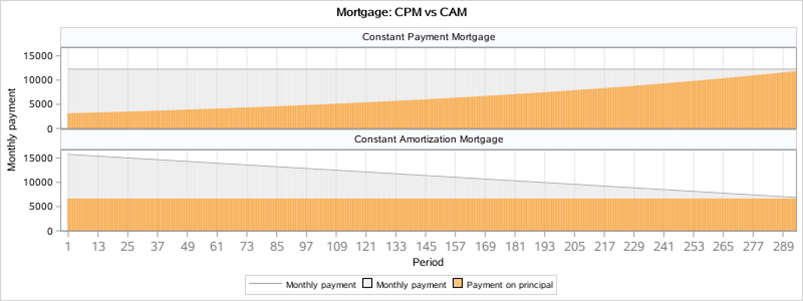Figure 4: Monthly payment of CPM vs CAM over period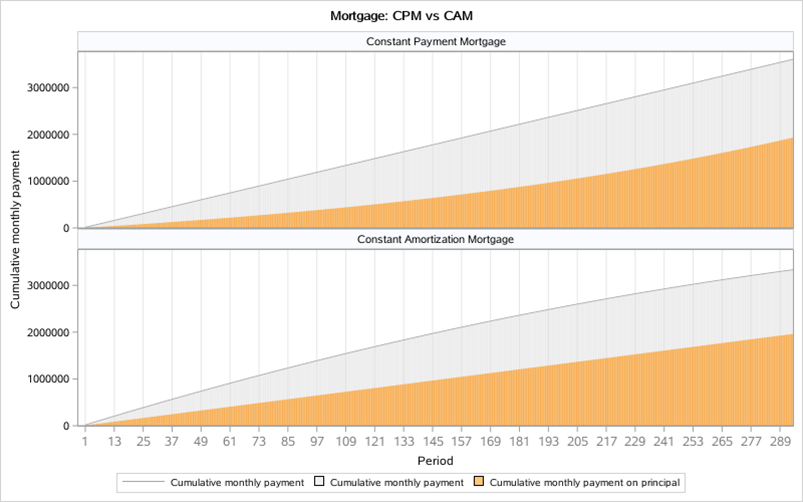Figure 5: Cumulative monthly payment of CPM vs CAM over period

Since the monthly payment of CPM is constant, the principal component increases month by month, but the interest component decreases accordingly. For CAM, the monthly payment and the interest component decreases rapidly month by month, but the principal component is constant.

CPM brings less stress to lenders and it is suitable for people whose income is expected to rise, and the lender is only willing to bear a lower monthly payment. CPM is also suitable for those people who have other channels to manage their own money to achieve greater benefits than mortgage expenditures. It is also suitable for those people who have a plan to sell real estate after a certain period. The disadvantage of CPM is that the overall interest expense is relatively high.

CAM is suitable for people who have higher income but whose income is flat or declining in the future. These people hope to put the payment stress on now rather than in the future. Declining payments may be appropriate to match a deflationary environment, but its high initial payment and rapid paydown of principal reduces leverage too fast. Furthermore, some people may complain the constantly changing payment is difficult to budget for and manage.

## Summary

We have summarized the design and implementation of SAS functions in financial calculations. We then provided examples to calculate and analyze the monthly payment, interest, and principal for CPM/CAM mortgages. Finally, we compared the monthly payment, principal, interest, and cumulative value changes to a period for two mortgage types.

Share